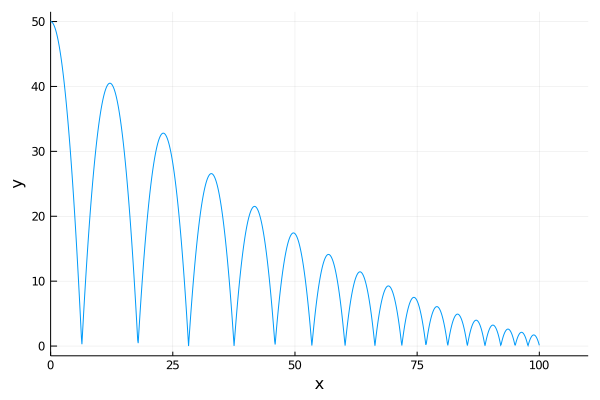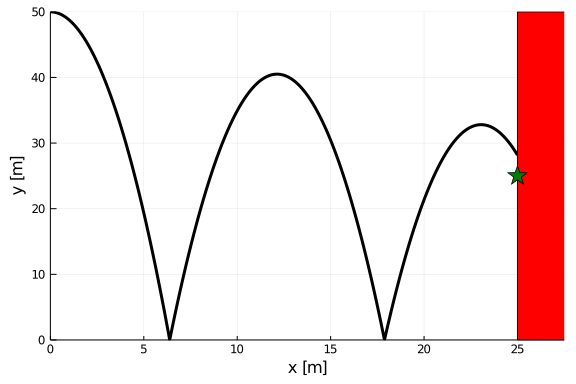# Optimization Under Uncertainty with DiffEqUncertainty.jl

This tutorial gives and overview of how to leverage the efficient Koopman expectation method from DiffEqUncertainty to perform optimization under uncertainty. We demonstrate this by using a bouncing ball model with an uncertain model parameter. We also demonstrate its application to problems with probabilistic constraints, in particular a special class of constraints called chance constraints.

## System Model

First lets consider a 2D bouncing ball, where the states are the horizontal position $x$, horizontal velocity $\dot{x}$, vertical position $y$, and vertical velocity $\dot{y}$. This model has two system parameters, acceleration due to gravity and coefficient of restitution (models energy loss when the ball impacts the ground). We can simulate such a system using ContinuousCallback as

using OrdinaryDiffEq, Plots

function ball!(du,u,p,t)
du = u
du = 0.0
du = u
du = -p
end

ground_condition(u,t,integrator) = u
ground_affect!(integrator) = integrator.u = -integrator.p * integrator.u
ground_cb = ContinuousCallback(ground_condition, ground_affect!)

u0 = [0.0,2.0,50.0,0.0]
tspan = (0.0,50.0)
p = [9.807, 0.9]

prob = ODEProblem(ball!,u0,tspan,p)
sol = solve(prob,Tsit5(),callback=ground_cb)
plot(sol, vars=(1,3), label = nothing, xlabel="x", ylabel="y")For this particular problem, we wish to measure the impact distance from a point $y=25$ on a wall at $x=25$. So, we introduce an additional callback that terminates the simulation on wall impact.

stop_condition(u,t,integrator) = u - 25.0
stop_cb = ContinuousCallback(stop_condition, terminate!)
cbs = CallbackSet(ground_cb, stop_cb)

tspan = (0.0, 1500.0)
prob = ODEProblem(ball!,u0,tspan,p)
sol = solve(prob,Tsit5(),callback=cbs)


To help visualize this problem, we plot as follows, where the star indicates a desired impace location

rectangle(xc, yc, w, h) = Shape(xc .+ [-w,w,w,-w]./2.0, yc .+ [-h,-h,h,h]./2.0)

begin
plot(sol, vars=(1,3), label=nothing, lw = 3, c=:black)
xlabel!("x [m]")
ylabel!("y [m]")
plot!(rectangle(27.5, 25, 5, 50), c=:red, label = nothing)
scatter!(,,marker=:star, ms=10, label = nothing,c=:green)
ylims!(0.0,50.0)
end## Considering Uncertainty

We now wish to introduce uncertainty in p, the coefficient of restitution. This is defined via a continuous univiate distribution from Distributions.jl. We can then run a Monte Carlo simulation of 100,000 trajectories via the EnsembleProblem interface.

using Distributions

cor_dist = truncated(Normal(0.9, 0.02), 0.9-3*0.02, 1.0)
trajectories = 100000

prob_func(prob,i,repeat) = remake(prob, p = [p, rand(cor_dist)])
ensemble_prob = EnsembleProblem(prob,prob_func=prob_func)